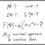Induction?

The question is quite famous and is generally included under the topic of induction, but I didn't actually catch how to do it. And it goes as follows:

Two sequences $(a_{1},a_{2},...)$ and $(b_{1},b_{2},...)$ are defined as $a_{1}=3, a_{n}=3^{a_{n-1}}$ and $b_{1}=4, b_{n}=4^{b_{n-1}}$. Show that $a_{1000}>b_{999}$.

Thank you to everyone who shall participate in the discussion and help me. Please explain how to do it, as well.Note by Shourya Pandey
6 years, 4 months ago

This discussion board is a place to discuss our Daily Challenges and the math and science related to those challenges. Explanations are more than just a solution — they should explain the steps and thinking strategies that you used to obtain the solution. Comments should further the discussion of math and science.

When posting on Brilliant:

• Use the emojis to react to an explanation, whether you're congratulating a job well done , or just really confused .
• Ask specific questions about the challenge or the steps in somebody's explanation. Well-posed questions can add a lot to the discussion, but posting "I don't understand!" doesn't help anyone.
• Try to contribute something new to the discussion, whether it is an extension, generalization or other idea related to the challenge.
• Stay on topic — we're all here to learn more about math and science, not to hear about your favorite get-rich-quick scheme or current world events.

MarkdownAppears as
*italics* or _italics_ italics
**bold** or __bold__ bold

- bulleted
- list

• bulleted
• list

1. numbered
2. list

1. numbered
2. list
Note: you must add a full line of space before and after lists for them to show up correctly
paragraph 1

paragraph 2

paragraph 1

paragraph 2

> This is a quote
This is a quote
# I indented these lines
# 4 spaces, and now they show
# up as a code block.

print "hello world"
# I indented these lines
# 4 spaces, and now they show
# up as a code block.

print "hello world"
MathAppears as
Remember to wrap math in $$...$$ or $...$ to ensure proper formatting.
2 \times 3 $2 \times 3$
2^{34} $2^{34}$
a_{i-1} $a_{i-1}$
\frac{2}{3} $\frac{2}{3}$
\sqrt{2} $\sqrt{2}$
\sum_{i=1}^3 $\sum_{i=1}^3$
\sin \theta $\sin \theta$
\boxed{123} $\boxed{123}$

Sort by:

First, you should prove that $a_{n}>b_{n-1}$ using induction. For the base case, let $n=2$, and it is obvious that $a_{n}=a_2=3^{a_1}=3^3=27$ is greater than $b_{n-1}=b_1=4$.

Now we must show that $a_{n+1}>b_n$ if we assume that $a_n>b_{n-1}$. $a_{n+1}=3^{a_n}$ and b_n=4^{b_{n-1}}. \(3^{a_n}>4^{b_{n-1}}

$\log_4{3^{a_n}}>b_{n-1}$

$a_n \cdot \log_4{3} > b_{n-1}$

$\log_4{3}>\frac{b_{n-1}}{a_n}$

This is the best I could do. I'm not sure how to proceed from here.

- 6 years, 4 months ago

I had proceeded in a similar manner, but to no avail. Maybe we need to prove it for certain powers or pattern and complete the proof by reverse induction, because I am sure that reverse induction works. But a reference point is what is required to be found.

- 6 years, 4 months ago

That's is a good start. However, as you realized, you needed to use a statement that is stronger than the induction hypothesis that you currently have. That factor of $\log_4 3$ seems to mess things up.

This suggests that you should make your induction hypothesis stronger, which was what Sebastian was doing.

For example, if the induction hypothesis was that $a_{n+1} > 2 b_n$, then you can see that the previous statement we wanted is true. However, of course, this strengthen the inequality that we get in the induction step, and hence may longer be true. Thus part of this problem is to find the stronger induction statement to prove.

I call this method of induction proof "stronger induction" (this terminology is not standard), because you have to guess at a stronger statement to show than the obvious guess. I am planning a series of posts on induction, and hope to cover this at some point in the future.

Staff - 6 years, 4 months ago

Well so the "stronger induction" here refers to the "stronger inequality" which is being used to prove the statement true.

- 6 years, 4 months ago

The word stronger refers to proving a stronger statement, then that given by the (initial) induction hypothesis. In this particular case, it is a stronger inequality.

Another example of a question approached by "stronger induction" is as follows:

$a, b$ are positive integers such that $\frac {2ab}{a^2+b^2} = \sin \theta$, show that $(a^2+b^2)^n \sin n\theta$ is an integer.

For this example, the induction hypothesis of

Let $P_n$ be the proposition that $(a^2+b^2)^n \sin n\theta$ is an integer.

is not sufficient enough for us to work on the induction step.

Staff - 6 years, 4 months ago